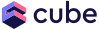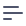0.33.23

# Getting started with data modeling

Data Modeling Workshop: Enhance Your Data Modeling Skills With Cube

Learn how Cube's data modeling makes it easier to build maintainable semantic layers with best practices & key improvements at our webinar on May 31st, 2023.

A Cube data model is used to transform raw data into meaningful business definitions and pre-aggregate data for optimal results. The data model is exposed through the querying API that allows end-users to query a wide variety of analytical queries without modifying the data model itself.

Let’s use a users table with the following columns as an example:

idpayingcitycompany_name
1trueSan FranciscoPied Piper
2truePalo AltoRaviga
3trueRedwoodAviato
4falseMountain ViewBream-Hall
5falseSanta CruzHooli

• How many users do we have?
• How many paying users?
• What is the percentage of paying users out of the total?
• How many users, paying or not, are from different cities and companies?

We don’t need to write SQL queries for every question, since the data model allows building well-organized and reusable SQL.

## 1. Creating a Cube

In Cube, cubes are used to organize entities and connections between entities. Usually one cube is created for each table in the database, such as users, orders, products, etc. In the sql_table parameter of the cube we define a base table for this cube. In our case, the base table is simply our users table.

YAML
JS
cubes:
- name: users
sql_table: users

## 2. Adding Measures and Dimensions

Once the base table is defined, the next step is to add measures and dimensions to the cube.

Measures are referred to as quantitative data, such as number of units sold, number of unique visits, profit, and so on.

Dimensions are referred to as categorical data, such as state, gender, product name, or units of time (e.g., day, week, month).

Let's go ahead and create our first measure and two dimensions:

YAML
JS
cubes:
- name: users
sql_table: users

measures:
- name: count
sql: id
type: count

dimensions:
- name: city
sql: city
type: string

- name: company_name
sql: company_name
type: string

Let's break down the above code snippet piece-by-piece. After defining the base table for the cube (with the sql_table property), we create a count measure in the measures block. The count type and sql id means that when this measure will be requested via an API, Cube will generate and execute the following SQL:

SELECT COUNT(id) AS count
FROM users;

When we apply a city dimension to the measure to see "Where are users based?", Cube will generate SQL with a GROUP BY clause:

SELECT city, COUNT(id) AS count
FROM users
GROUP BY 1;

You can add as many dimensions as you want to your query when you perform grouping.

## 3. Adding Filters to Measures

Now let's answer the next question – "How many paying users do we have?". To accomplish this, we will introduce measure filters:

YAML
JS
cubes:
- name: users

measures:
- name: count
sql: id
type: count

- name: paying_count
sql: id
type: count
filters:
- sql: "{CUBE}.paying = 'true'"

# ...

It is best practice to prefix references to table columns with the name of the cube or with the CUBE constant when referencing the current cube's column.

That's it! Now we have the paying_count measure, which shows only our paying users. When this measure is requested, Cube will generate the following SQL:

SELECT
COUNT(
CASE WHEN (users.paying = 'true') THEN users.id END
) AS paying_count
FROM users

Since the filters property is an array, you can apply as many filters as required. paying_count can be used with dimensions the same way as a simple count. We can group paying_count by city and companyName simply by adding these dimensions alongside measures in the requested query.

## 4. Using Calculated Measures

To answer "What is the percentage of paying users out of the total?", we need to calculate the paying users ratio, which is basically paying_count / count. Cube makes it extremely easy to perform this kind of calculation. Let's add a new measure to our cube called paying_percentage:

YAML
JS
cubes:
- name: users

measures:
- name: count
sql: id
type: count

- name: paying_count
sql: id
type: count
filters:
- sql: "{CUBE}.paying = 'true'"

- name: paying_percentage
sql: "100.0 * {paying_count} / {count}"
type: number
format: percent

# ...

Here we defined a calculated measure paying_percentage, which divides paying_count by count. This example shows how you can reference measures inside other measure definitions. When you request the paying_percentage measure via an API, the following SQL will be generated:

SELECT
100.0 * COUNT(
CASE WHEN (users.paying = 'true') THEN users.id END
) / COUNT(users.id) AS paying_percentage
FROM users

As with other measures, paying_percentage can be used with dimensions.

## 5. Next Steps